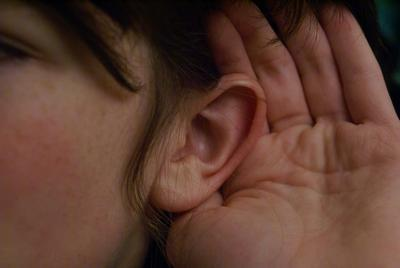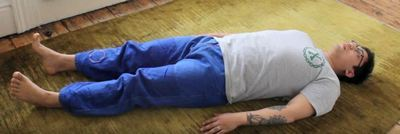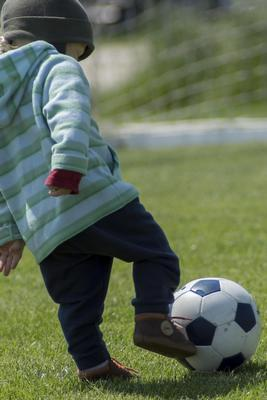Like   Tweet   Pin   +1   in
 /* styles */ Tai Chi Stories, Tips and Resources   ||   Issue #025 April 2020
 table div table+table+table div table{width:100%;padding:0}table div table+table+table div table img{width:96.23%;padding:0;float:none}table div table+table+table div table td{width:100%;padding:0 1.88% 18px}/* styles */In this issue I cover what I consider are the real secrets of Tai Chi.

There's also a Tai Chi tip, an interesting interpretation on Chi from Master Chia, a Lao Tzu quote, and a lying down Qigong clip that may be of interest to aged care staff. Plus a post related to sport, with a checklist for better training that applies to Tai Chi training as well.

Regards
Chris Bennett
Chris Chi

 table div table+table+table+table+table div table{width:100%;padding:0}table div table+table+table+table+table div table img{width:96.23%;padding:0;float:none}table div table+table+table+table+table div table td{width:100%;padding:0 1.88% 18px}/* styles */## The Three Secrets of Tai ChiI never thought I'd use the word "secrets" in a post about Tai Chi because it's usually marketing hype. But essentially I'm breaking my rule here.

I'm always sceptical when I see the word "secret" added to a title of a Tai Chi or Chi Kung book, post, or a video.

(Image courtesy of JPPI via Morgue File)

 table div table+table+table+table+table+table+table+table div table{width:100%;padding:0}table div table+table+table+table+table+table+table+table div table img{width:96.23%;padding:0;float:none}table div table+table+table+table+table+table+table+table div table td{width:100%;padding:0 1.88% 18px}/* styles */## Tai Chi Tip

 /* styles */ When standing in a Tai Chi posture avoid rolling your hips forward
 table div table+table+table+table+table+table+table+table+table+table+table div table{width:100%;padding:0}table div table+table+table+table+table+table+table+table+table+table+table div table img{width:96.23%;padding:0;float:none}table div table+table+table+table+table+table+table+table+table+table+table div table td{width:100%;padding:0 1.88% 18px}/* styles */## Chi Is Bio Magnetic Electric EnergyIn this 4.59 clip Master Chia talks about Chi as bio magnetic electric energy.

He covers themes on 'aligning energy around you, using inexhaustable energy, focus and concentrated energy'.

 table div table+table+table+table+table+table+table+table+table+table+table+table+table+table div table{width:100%;padding:0}table div table+table+table+table+table+table+table+table+table+table+table+table+table+table div table img{width:96.23%;padding:0;float:none}table div table+table+table+table+table+table+table+table+table+table+table+table+table+table div table td{width:100%;padding:0 1.88% 18px}/* styles */## Lying Down Qigong and Tai Chi ChuanThis is a long clip atb22.47 minutes. But worth watching if you want to practice Qigong lying down on the floor or in bed. It's from Acupunx and contains several short segments of breathing and moving exercises.

I may develop my own staff training program so staff can teach aged care residents with restricted mobility issues, in the aged care sector - if there is interest.

 table div table+table+table+table+table+table+table+table+table+table+table+table+table+table+table+table+table div table{width:100%;padding:0}table div table+table+table+table+table+table+table+table+table+table+table+table+table+table+table+table+table div table img{width:96.23%;padding:0;float:none}table div table+table+table+table+table+table+table+table+table+table+table+table+table+table+table+table+table div table td{width:100%;padding:0 1.88% 18px}/* styles */## Ten Tips for Sports TrainingElizabeth Quinn's, Athlete's Checklist for Better Training post applies to Tai Chi training as well.

Her ten tips are guidelines I always try and follow when I do Tai Chi training.

The one tip I have to be careful with is over training because I'm tempted to do more than I can handle.

(Image courtesy of GaborFromHungary (via Morgue File)

 table div table+table+table+table+table+table+table+table+table+table+table+table+table+table+table+table+table+table+table+table div table{width:100%;padding:0}table div table+table+table+table+table+table+table+table+table+table+table+table+table+table+table+table+table+table+table+table div table img{width:96.23%;padding:0;float:none}table div table+table+table+table+table+table+table+table+table+table+table+table+table+table+table+table+table+table+table+table div table td{width:100%;padding:0 1.88% 18px}/* styles */table div table+table+table+table+table+table+table+table+table+table+table+table+table+table+table+table+table+table+table+table+table+table div table{width:100%;padding:0}table div table+table+table+table+table+table+table+table+table+table+table+table+table+table+table+table+table+table+table+table+table+table div table img{width:96.23%;padding:0;float:none}table div table+table+table+table+table+table+table+table+table+table+table+table+table+table+table+table+table+table+table+table+table+table div table td{width:100%;padding:0 1.88% 18px}/* styles *//* styles */
 table div table+table+table+table+table+table+table+table+table+table+table+table+table+table+table+table+table+table+table+table+table+table+table+table div table{width:100%;padding:0}table div table+table+table+table+table+table+table+table+table+table+table+table+table+table+table+table+table+table+table+table+table+table+table+table div table img{width:96.23%;padding:0;float:none}table div table+table+table+table+table+table+table+table+table+table+table+table+table+table+table+table+table+table+table+table+table+table+table+table div table td{width:100%;padding:0 1.88% 18px}/* styles *//* styles */